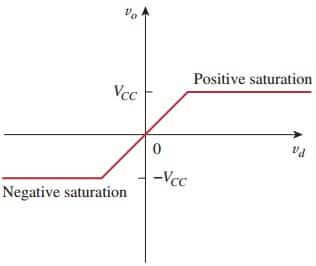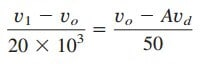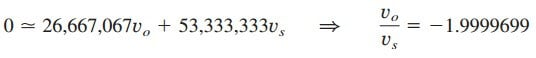# Short Definition: What is Operational AmplifierDo you know what is operational amplifier? Having learned the basic laws and theorems for circuit analysis, we are now ready to study an active circuit element of paramount importance: what is operational amplifier, or op amp for short. The op amp is a versatile circuit building block.

The op amp is an electronic unit that behaves like a voltage- controlled voltage source.

It can also be used in making a voltage- or current -controlled current source. An op amp can sum signals, amplify a signal, integrate it, or differentiate it. The ability of the op amp to perform these mathematical operations is the reason it is called an operational amplifier.

It is also the reason for the widespread use of op amps in analog design. Op amps are popular in practical circuit designs because they are versatile, inexpensive, easy to use, and fun to work with.

We begin by discussing the ideal op amp and later consider the non-ideal op amp. Using nodal analysis as a tool, we consider ideal op amp circuits such as the inverter, voltage follower, summer, and difference amplifier.

We will also analyze op amp circuits with PSpice. Finally, we learn how an op amp is used in digital-to-analog converters and instrumentation amplifiers.

## What is Operational Amplifiers

An operational amplifier is designed so that it performs some mathematical operations when external components, such as resistors and capacitors, are connected to its terminals. Thus,

An op amp is an active circuit element designed to perform mathematical operations of addition, subtraction, multiplication, division, differentiation, and integration.

The op amp is an electronic device consisting of a complex arrangement of resistors, transistors, capacitors, and diodes. A full discussion of what is inside the op amp is beyond the scope of this post. It will suffice to treat the op amp as a circuit building block and simply study what takes place at its terminals.

Op amps are commercially available in integrated circuit packages in several forms. Figure.(1) shows a typical op amp package.

A typical one is the eight-pin dual in-line package (or DIP), shown in Figure.(2a).

Pin or terminal 8 is unused, and terminals 1 and 5 are of little concern to us. The five important terminals are:

1. The inverting input, pin 2.
2. The noninverting input, pin 3.
3. The output, pin 6.
4. The positive power supply V+, pin 7.
5. The negative power supply V , pin 4.

The circuit symbol for the op amp is the triangle in Figure.(2b); as shown, the op amp has two inputs and one output. The inputs are marked with minus () and plus (+) to specify inverting and noninverting inputs, respectively.

An input applied to the noninverting terminal will appear with the same polarity at the output, while an input applied to the inverting terminal will appear inverted at the output.

As an active element, the op amp must be powered by a voltage supply as typically shown in Figure.(3).

Although the power supplies are often ignored in op amp circuit diagrams for the sake of simplicity, the power supply currents must not be overlooked.

By KCL,

The equivalent circuit model of an op amp is shown in Figure.(4).

The output section consists of a voltage-controlled source in series with the output resistance Ro. It is evident from Figure.(4) that the input resistance Ri is the Thevenin equivalent resistance seen at the input terminals, while the output resistance Ro is the Thevenin equivalent resistance seen at the output. The differential input voltage vd is given by

where v1 is the voltage between the inverting terminal and ground and v2 is the voltage between the noninverting terminal and ground. The op amp senses the difference between the two inputs, multiplies it by the gain A, and causes the resulting voltage to appear at the output.

Thus, the output vo is given by

A is called the open-loop voltage gain because it is the gain of the op amp without any external feedback from output to input. Table.(1) shows typical values of voltage gain A, input resistance Ri, output resistance Ro, and supply voltage VCC.

The concept of feedback is crucial to our understanding of op amp circuits. Negative feedback is achieved when the output is fed back to the inverting terminal of the op amp.

As Example.(1) below shows, when there is a feedback path from output to input, the ratio of the output voltage to the input voltage is called the closed-loop gain.

As a result of the negative feedback, it can be shown that the closed-loop gain is almost insensitive to the open-loop gain A of the op amp. For this reason, op amps are used in circuits with feedback paths.

A practical limitation of the op amp is that the magnitude of its output voltage cannot exceed |VCC|. In other words, the output voltage is dependent on and is limited by the power supply voltage.

Figure.(5) illustrates that the op amp can operate in three modes, depending on the differential input voltage vd:

1. Positive saturation, vo = VCC.
2. Linear region, VCC vo = Avd VCC.
3. Negative saturation, vo = –VCC.Figure 5. Op amp output voltage vo as a function of the differential input voltage vd

If we attempt to increase vd beyond the linear range, the op amp becomes saturated and yields vo = VCC or vo = −VCC. Throughout this book, we will assume that our op amps operate in the linear mode. This means that the output voltage is restricted by

Although we shall always operate the op amp in the linear region, the possibility of saturation must be borne in mind when one designs with op amps, to avoid designing op amp circuits that will not work in the laboratory.

After learning what is operational amplifier, we will also learn:

## Operational Amplifier Circuit Example

A 741 op amp has an open-loop voltage gain of 2 × 105, the input resistance of 2 MΩ, and output resistance of 50Ω. The op amp is used in the circuit of Figure.(6a). Find the closed-loop gain vo/vs. Determine current i when
vs =2 V.

Solution:
Using the op amp model in Figure.(4), we obtain the equivalent circuit of Figure.(6a) as shown in Figure.(6b). We now solve the circuit in Fig. 5.6(b) by using nodal analysis.

At node 1, KCL givesMultiplying through by 2000 × 103, we obtain

At node O,But vd = −v1 and A = 200,000. ThenThis is closed-loop gain because the 20 kΩ feedback resistor closes the loop between the output and input terminals.